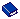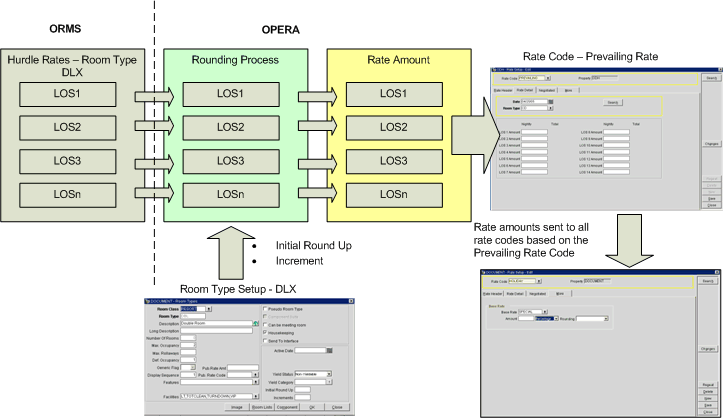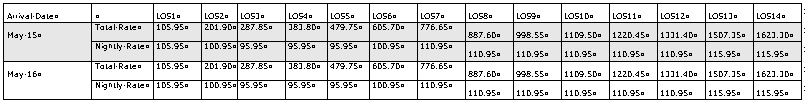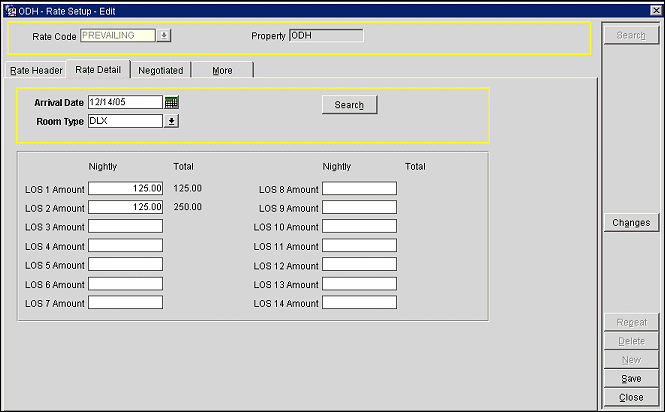Rates by Day by Room Type by LOS

Note: Available only when the OPP_OPUS OPERA Revenue Management add on license is active.

With the activation of the Rates>Prevailing Rate by LOS application function, OPERA can create daily rate amounts per room type per length of stay based on bid prices/hurdle rates sent down by ORMS and other yield management systems.

When the Rates>Prevailing Rate by LOS application function is set to Y, the Rates>Prevailing Rate Code application setting becomes active. The prevailing rate code identified by this setting tells OPERA which rate code will hold the rate amounts (prevailing rates) created or updated based on the length of stay hurdle/bid prices from ORMS.  The prevailing rates will be calculated automatically and dynamically by OPERA's Rates by Day by Room Type by LOS process. For this reason, in order for a rate code to be selected as the prevailing rate code via the Rates>Prevailing Rate Code application setting, the rate code must meet the following criteria:

• It must not have rate details configured.
• If the Rates>Rate Tiers application function is set to Y for the property, the rate code must not be flagged as Tiered.
• The rate code must be of the same currency as the Yield Currency Code, set by the General>Yield Currency Code application setting, available when the OPP_OPUS OPERA Revenue Management System license code is active. If the property is not using the OPP_OPUS license, the rate code must be of the same currency as the property's default currency.

Note: If the Rates>Prevailing Rate by LOS application function is set to Y, a specialized Rate Query Details screen displays the prevailing rates for the guest's requested arrival date, plus 3 days prior to and three days following the arrival date. See Prevailing Rate Query for details. Prevailing Rates can also be displayed on the ORS Sales Screen|Rate Availability Screen as the Default Display Set. See ORS Sales Screen for more information.

Each day ORMS sends hurdle rates for all room types that are "yieldable." Hurdle rates are sent for a range of Length of Stays. After calculation, the prevailing rate code will hold rate amounts per day per LOS for all room types sent from ORMS. Up to 15 other rate codes can be based on the prevailing rate code (by selecting the prevailing rate code as the Base Rate on the Rate Setup screen More tab); rate amounts for these other rate codes will be driven by the rate amounts calculated for the prevailing rate code.### Rate Calculation Methodology

The above diagram shows the major elements in the prevailing rate amount calculation process. ORMS sends daily hurdle rates to OPERA for each yieldable room type and length of stay for a date. The rounding process takes the daily hurdle rate for each room type/LOS and calculates the prevailing rate amount for the day by room type and LOS using the Rounding and Increment values for the applicable room type. The rounding and increment processing would be applicable to all room types associated with a yield category.

The prevailing rate amount calculations are made in the following manner.

1. The hurdle rate amount for each room type per LOS is divided by the number of nights. For example, if the 2-night hurdle rate for the DLX room type is 208.50, the calculated nightly rate is 104.25.
2. The rounding process checks the Room Type Setup for each yieldable room type. Two values are taken from the Room Type Setup: the initial round up value and the increment value.
• Initial Round Up - A number which is greater than 0.00, but not more than 50.00. The digits of the initial round up value replace the last two whole numbers and any decimals of the calculated nightly rate. For example, if the calculated nightly rate is 104.25 and the initial round up is 4.95, the calculated nightly rate becomes 104.95 after the initial round up is applied. (If the calculated nightly rate were 114.25 and the initial round up is 4.95, the calculated nightly rate would also become 104.95.)
• Increment - A whole number (no decimals — for example, 5, 6, 10, 23 ) which must be greater than or equal to the initial round up amount. The sum of the increment and the initial round up cannot be greater than 100 (e.g., if the initial round up is 39.95, then the increment could not be greater than 60).  The increment is added to the calculated nightly rate after the initial round up is applied if the calculated nightly rate amount is less than the hurdle rate. For example, if the hurdle rate is 107.25 and the initial round up is 4.95, the calculated nightly rate becomes 104.95 after the initial round up is applied. This is less than the hurdle rate so the increment, 5, is added to the calculated nightly rate amount to make it 109.95. The increment can be added multiple times, if necessary, to make the calculated nightly rate equal to, or greater than, the hurdle rate.
3. After rounding, the calculated nightly rate is multiplied by the number of nights for the LOS to obtain the prevailing rate amount for the total stay. This rate amount is the prevailing rate amount for the day by room type by LOS. Prevailing rates may be passed to a maximum of 15 rate codes based on the prevailing rate code.

The following table gives additional examples of the rounding process.

 Hurdle Rate Initial Round Up Increment Prevailing Rate Amount 104.25 4.95 5 104.95 107.25 4.95 5 109.95 111.25 4.95 5 114.95 125.50 4.95 5 129.95 101.02 0.04 5 105.04 101.25 0.04 5 105.04 302.50 20.00 25 320.00 321.20 20.00 25 345.00 350.00 20.00 25 370.00

The last example in the above table shows that an increment can be applied more than once, if necessary. The initial round up produces a rate amount of 320. Because this is lower than the hurdle rate, the increment (25) is added, resulting in a rate amount of 345. This is still lower than the hurdle rate so the increment is applied again, resulting in a 370 prevailing rate amount.

Hurdle rates for LOS1 through LOS7 are passed to OPERA from TLP. OPERA computes rate amounts for LOSs of up to 14 nights. The rate amounts for the extra nights are calculated by taking the hurdle amount for the 7-night LOS plus the hurdle amounts for the applicable extra number of additional nights based on the extra number of nights' applicable hurdle value, and then rounding.

Example:

Here is a hypothetical example that illustrates the prevailing rate calculation process for a DLSV room type when the guest is arriving on 11/21/06 and staying for 14 nights.

1. The following LOS hurdle rates were received by the hotel:
• The LOS7 hurdle for room type DLSV was \$805 for 11/21/06.
• The LOS1 hurdle for room type DLSV was \$89 for 11/28/06.
• The LOS1 hurdle for room type DLSV was \$89 for 11/29/06.
• The LOS1 hurdle for room type DLSV was \$89 for 11/30/06.
• The LOS1 hurdle for room type DLSV was \$129 for 12/01/06.
• The LOS1 hurdle for room type DLSV was \$129 for 12/02/06.
• The LOS1 hurdle for room type DLSV was \$89 for 12/03/06.
• The LOS1 hurdle for room type DLSV was \$89 for 12/04/06.
2. The LOS hurdle rates are added and then divided by the number of nights to get a nightly rate:

805 +89 +89 +89 +129+129+89+89 = 1508

1508 /14 = 107.71

3. The rounding process is now applied to the calculated nightly rate. Room type DLSV has rounding set to 4.95, and an increment of 5.00.

Applying the rounding value to the 107.71 per-night rate results in 104.95.

104.95 is less than the 107.71 hurdle rate so the increment value for the room type, 5.00,  is applied.

104.95 + 5 = 109.95

4. The rate of 109.95 satisfies the hurdle and is the rate shown for the prevailing rate code DLSV in the rate query for a 14-night stay arriving on 11/21/06.

#### Rate Amount Storage in OPERA for Prevailing Rates

To enable quotes based on arrival date, LOS, and room type, rate storage for the prevailing rate code must be more extensive than for other OPERA rate codes. Amounts for stays up to 14 nights are stored. Stored rates for room type DLX for arrival dates May 15 and May 16 might be represented like this:### Prevailing Rate Code Configuration

To view the calculated LOS rate amounts by date and room type (the prevailing rates), select the Rate Detail tab on the Rate Setup screen (Configuration>Rate Management>Rate Classifications>Rate Codes) for the prevailing rate code (the rate code defined by the application setting, Rates>Prevailing Rate Code). You may edit these rate amounts manually, but any changes made will be overwritten when ORMS sends new hurdle rates and the Rates by Day by Room Type by LOS process recalculates rate amounts. When manually entering rates here, OPERA does not take into consideration the rounding and increment values — only the number of nights. For example, if the user enters the nightly rate amount for DLX for 01.01.10 for LOS 2 as 175.00, the total amount for a 2-night stay for the noted room type and date combination would be 350.00.### Rate Codes Based on the Prevailing Rate Code

A maximum of 15 rate codes may be based on the prevailing rate code. (A rate code may be based on another rate code by using the features provided on the Rate Code Setup screen More tab.) When configuring base rate functionality for a rate code, you can select the room types for which rates will be set by the base (prevailing) rate.  When a new room type is added to a rate code that is based on the prevailing rate, a rate amount will not be created until the next hurdle rate update is sent from TLP.

Base rate functionality allows either a flat amount (e.g., -10.00, 12.50) or a percentage (e.g., -5%, 10%) difference from the prevailing  rate amount.

Here are some examples showing how rate amounts are calculated.

 LOS Room Type Prevailing Rate Code Total Stay Amount Rate Code Based on Prevailing Rate Total Stay Amount Nightly Rate Amount LOS1 DLX 105.95 105.95/1 nts = 105.95 105.95 - 10% = 95.3595.35 * 1 nts = 95.36 95.36 LOS2 DLX 201.90 201.90/2 nts = 100.95100.95 - 10% = 90.8690.86 * 2 nts = 181.72 90.86 LOS3 DLX 287.85 287.85/3 nts = 95.9595.95 - 10% =86.3686.36 * 3 nts = 259.08 86.36

When the bid for an arrival date is changed, OPERA recalculates rate amounts for those rate codes that are based on the prevailing rate code for the affected room types.

Standard yield functionality compares the hurdle rate to the rate amount determined by the rate code specifications in order to determine availability. The Yield As field on the Rate Code Setup screen header  allows you to specify a rate code to which the current rate will defer should it happen to fall below the hurdle rate. For rate codes based on the prevailing rate code, it is assumed that the Yield As option on the Rate Code Setup screen header will be set to the prevailing rate code. This will prevent the possibility of its coming in lower than the hurdle rate and therefore being closed.

It is also understood that rate codes based on the prevailing rate must be manually opened and closed should their availability need to be restricted.

### Room Type Configuration

The Room Type screen (Configuration>Reservations>Room Classifications>Room Types) includes the Initial Round Up and Increments fields when the Rates>Prevailing Rate by LOS application function is set to Y. These fields provide inputs to the Rate by Day by Room Type by LOS rounding process which are specific to the room type being processed. These fields are described above in Rate Calculation Methodology.

Note: In Multi-property environments, Initial Round Up and Increments may be configured at the individual property level only. These fields are not available when creating or editing room type templates.